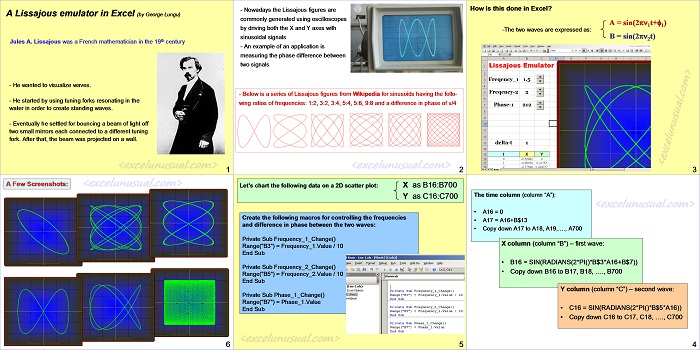# Tutorial – a Static Lissajous Emulator

Hi there, this is a tutorial explaining the construction of a very basic Lissajous emulator in Excel.

It’s supposed to be very easy to understand at the high school level.

Good luck,

George[sociallocker][/sociallocker]

## A Lissajous emulator in Excel

by George Lungu

### Introduction:

Jules A. Lissajous was a French mathematician from the 19th century

– He wanted to visualize waves.

– He started by using tuning forks resonating in the water in order to create standing waves.

– Eventually he settled for bouncing a beam of light off two small mirrors each connected to a different tuning fork. After that, the beam was projected on a wall.

– Nowadays the Lissajous figures are commonly generated using oscilloscopes by driving both the X and Y axes with sinusoidal signals.

– An example of an application is measuring the phase difference between two signals.

– Below is a series of Lissajous figures from Wikipedia for sinusoidal signals having the follo-wing ratios of frequencies: 1:2, 3:2, 3:4, 5:4, 5:6, 9:8 and a difference in phase of pi/4.

### Implementation – How is this done in Excel?

#### -The two waves are expressed as:

A = sin(2pn1t+f1)
B = sin(2pn2t)

<www.excelunusual.com> 3

The time column (column “A”):

• A16 = 0
• A17 = A16+B\$13
• Copy down A17 to A18, A19,…., A700

X column (column “B”) – first wave:

• Copy down B16 to B17, B18, …., B700

Y column (column “C”) – second wave:

• Copy down C16 to C17, C18, …., C700

<www.excelunusual.com> 4

X as B16:B700
Y as C16:C700

#### Create three macros for controlling the frequencies and difference in phase between the two waves:

Private Sub Frequency_1_Change()
Range(“B3”) = Frequency_1.Value / 10
End Sub

Private Sub Frequency_2_Change()
Range(“B5”) = Frequency_2.Value / 10
End Sub

Private Sub Phase_1_Change()
Range(“B7”) = Phase_1.Value
End Sub
5

A Few Screenshots:

– 1.5 -1 -0.5 0 0.5 1 1.5
0
– 1.5 -1 -0.5 0 0.5 1 1.5
0
– 1.5 -1 -0.5 0 0.5 1 1.5

by George Lungu <www.excelunusual.com>